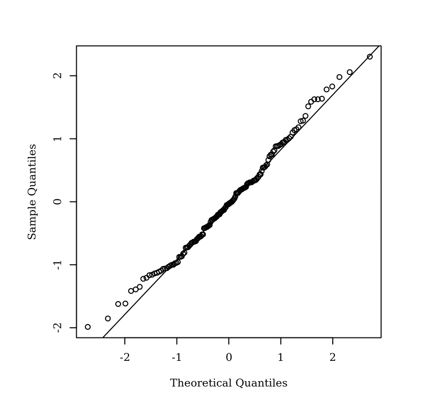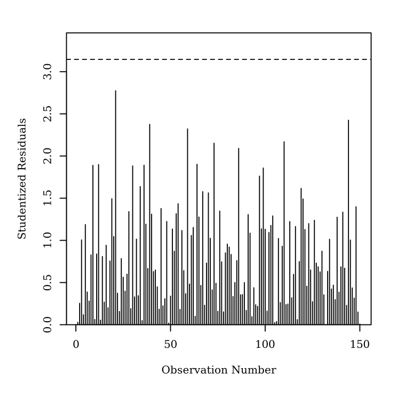# Why would you conduct a linear regression?

Posted July 14, 2020

The linear regression is a test to see if predictors can explain an outcome variable.  For example, do two predictors (e.g., Stress Level and college GPA) predict an outcome variable (e.g., Life Satisfaction).  The results of the regression will indicate whether the model (using stress and GPA) are good at assessing Satisfaction, which of these predictors were important in predicting satisfaction, and what was the relationship between a predictor and the outcome variable.

### Assumptions

The linear regression has the assumptions of normality, homoscedasticity, multicollinearity, and the absence of outliers.

The assumption of normality can be assessed by plotting the quantiles of the model residuals against the quantiles of a Chi-square distribution, also called a Q-Q scatterplot. For the assumption of normality to be met, the quantiles of the residuals must not strongly deviate from the theoretical quantiles. Strong deviations indicate that the parameter estimates are unreliable. Below is an example plot.Homoscedasticity can be evaluated by plotting the residuals against the predicted values. The assumption of homoscedasticity is met if the points appear randomly distributed with a mean of zero and no apparent curvature. Below is an example plot.Variance Inflation Factors (VIFs) are calculated to detect the presence of multicollinearity (high correlations) between predictor variables.  High VIFs (typically greater than 5) indicates increased effects of multicollinearity in the model.

To identify outliers or influential points, Studentized residuals can be calculated and the absolute values were plotted against the observation numbers. Studentized residuals are calculated by dividing the model residuals by the estimated residual standard deviation. An observation with a Studentized residual greater than 3.15 in absolute value, the 0.999 quartile of a t distribution with 149 degrees of freedom, is considered to have significant influence on the results of the model. Below is an example plot.The results of a linear regression

After assessing the assumptions, the regression will indicate if the model was significant, indicated by a statistically significant F-value.  The coefficient of determination will be shown in the R-squared value.  Each predictor will have a beta coefficient, a t-value, and a probability for that t-value (a probability of 0.05 or less is considered statistically significant). The beta sign (positive or negative) indicates the relationship between that predictor and the outcome variable.  For example, if stress has a negative beta coefficient, this indicates that the higher the stress, the lower the satisfaction tends to be, while a positive beta for GPA indicates that the higher the GPA, the higher the satisfaction tends to be.

If you want to see for yourself, you can go to www.IntellectusStatistics.com, try it for a week for free, download an example dataset and conduct a linear regression on different data, and look at the results.

Shares